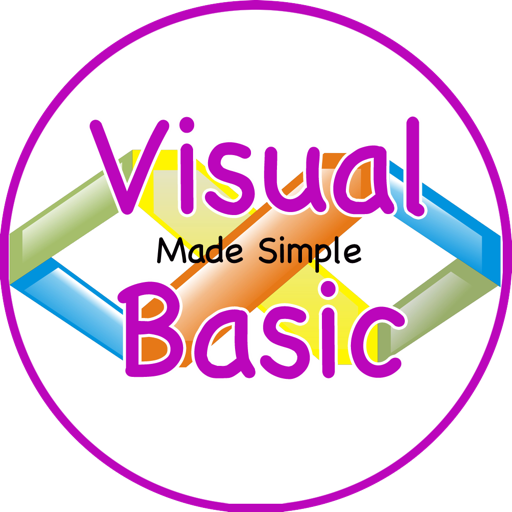Made Simple! For Visual Basic# Made Simple! For Visual Basic

## Tony Walsh

4.1 for iPhone
\$6.99
0

0 Ratings

Update Date

2017-09-27

Size

1.9 MB

Made Simple! For Visual Basic Description
Visual Basic (VB) is a programming environment from Microsoft in which a programmer uses a graphical user interface (GUI) to choose and modify preselected sections of code written in the BASIC programming language.

Based on the BASIC language, Visual Basic was one of the first products to provide a graphical programming environment and a paint metaphor for developing user interfaces. Instead of worrying about syntax details, the Visual Basic programmer can add a substantial amount of code simply by dragging and dropping controls, such as buttons and dialog boxes, and then defining their appearance and behavior.
Although not a true object-oriented programming language in the strictest sense, Visual Basic nevertheless has an object-oriented philosophy. It is sometimes called an event-driven language because each object can react to different events such as a mouse click.

This collection of 200 Video Tutorial Lessons Will soon get you up to speed.

Use this app as a “Video Referencing Library” where you can come back for a refresher lesson or search for how to do something new.

App features:
** Edit the video title, subtitle and keep your own set of user notes.
** Move the video around it's group and even change it's group.
** Search by title or notes
** View by favourites or rating
** View by history the last ten played or visited videos

Lessons include:
Visual Basic Tutorial - 1 - What Is Visual Basic
Visual Basic Tutorial - 2 - Installing The Visual Basic IDE
Visual Basic Tutorial - 3 - Getting Familiar With The IDE
Visual Basic Tutorial - 4 - Hello World
Visual Basic Tutorial - 5 - Primitive Data Types
Visual Basic Tutorial - 6 - Comments And Whitespace
Visual Basic Tutorial - 7 - Errors
Visual Basic Tutorial - 8 - Variables
Visual Basic Tutorial - 9 - Math Operators
Visual Basic Tutorial - 10 - More On Math Operators
Visual Basic Tutorial - 11 - Getting User Input
Visual Basic Tutorial - 12 - Creating A Basic Calculator
Visual Basic Tutorial - 13 - If Statement
Visual Basic Tutorial - 14 - Else If And Else
Visual Basic Tutorial - 15 - Nested If Statements
Visual Basic Tutorial - 16 - Conditional Operators
Visual Basic Tutorial - 17 - Logical Operators
Visual Basic Tutorial - 18 - Concatenate Strings
Visual Basic Tutorial - 19 - Get Length Of Strings
Visual Basic Tutorial - 20 - SubStrings
Visual Basic Tutorial - 21 - Formatting Strings
Visual Basic Tutorial - 22 - Replacing SubStrings
Visual Basic Tutorial - 23 - Comparing Strings
Visual Basic Tutorial - 24 - Select Case
Visual Basic Tutorial - 25 - Select Case Else
Visual Basic Tutorial - 26 - For Next Loop
Visual Basic Tutorial - 27 - Step Operator
Visual Basic Tutorial - 28 - Exiting For Loop
Visual Basic Tutorial - 29 - Continue For
Visual Basic Tutorial - 30 - Do Until Loop
Visual Basic Tutorial - 31 - Do While Loop
Visual Basic Tutorial - 32 - Exit Do Loops
Visual Basic Tutorial - 33 - More On Do Loops
Visual Basic Tutorial - 34 - Nested Loops
Visual Basic Tutorial - 35 - The Infinte Loop
Visual Basic Tutorial - 36 - Introduction To Windows Forms
Visual Basic Tutorial - 37 - Form Properties
Visual Basic Tutorial - 38 - ToolBox
Visual Basic Tutorial - 39 - MessageBoxes
Visual Basic Tutorial - 40 - MessageBox Input
Visual Basic Tutorial - 41 - Input Box
Visual Basic Tutorial - 42 - User Defined Subs
Visual Basic Tutorial - 43 - Functions
Visual Basic Tutorial - 44 - ByVal
Visual Basic Tutorial - 45 - ByRef
Visual Basic Tutorial - 46 - Optional ByVal
Visual Basic Tutorial - 47 - Coercion
Visual Basic Tutorial - 48 - Exit Subs
Visual Basic Tutorial - 49 - Events
Visual Basic Tutorial - 50 - Global Variables
Visual Basic Tutorial - 51 - Constants
Visual Basic Tutorial - 52 - Math Class
Visual Basic Tutorial - 53 - CType
Visual Basic Tutorial - 54 - Calculator Part 1

and more.
Made Simple! For Visual Basic 4.1 Update
2017-09-27 Version History
1.Search Bug repaired
3.Full Play Screen Enabled
More